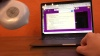Activity 4: Position Recognition (Python)

In the previous activities, you used the Google Teachable Machine tools to create a machine learning model. You couldn’t really see much of the math that is happening behind the scenes to determine which class a sample belongs to. In this activity, you will create a simple machine learning model yourself to get an idea of what is going on behind the scenes.

This activity consists of several steps that will guide you through implementing what is known in machine learning as a nearest-neighbor algorithm.

• Collect training data.
• Write a function to quantify the distance between a training sample and a test sample.
• Predict the class of a test sample by finding which of the training samples is closest to it, which is known as its “nearest neighbor.”This file already contains a function to collect training data. You will collect acceleration data from the Finch in three different positions. You must hold the Finch in each position for about five seconds. Run the file to try it out. After it collects the training data, the program will print the first training sample in the console as an example. Each training sample is a list that consists of the acceleration of the Finch in x, y, and z directions, as well as the class label.Quantifying Distance Between SamplesThe goal of the nearest neighbor algorithm is to find the training sample that is “closest” to a test sample. Once you find this nearest neighbor, you predict that the test sample will have the same class as that neighbor.The first step is to calculate the difference between a training sample and a test sample. There are a lot of ways to do this, but we will use the Euclidean distance. This is the same way that you find the distance between two points (a, b) and (c, d) on the coordinate grid. The distance between the two points is the square root of (ac)2 + (bd)2. We will use the same method, except that the samples may have more than two coordinates.

Start by defining a function named distanceBetween() that takes two parameters, trainingSample and testSample. Define a variable named squaredDistance that is equal to 0.For each of the values in testSample, calculate the squared difference between that value and the corresponding value in trainSample, then add it to squaredDifference. Notice that we are using the length of testSample in our for loop. trainingSample has one additional value (the class label) that should not be included in this computation.Predicting the Class of a Test SampleThe next step in building your model is to use your distanceBetween() function to compare a new test sample to each of the training samples in order to find the nearest neighbor. You will find the distance between the test sample and each training sample. The training sample with the smallest distance is the nearest neighbor. In this diagram, the nearest neighbor is the circled orange point.Declare a function named getPrediction(). This function will take two parameters. The first is trainingSet, which is the data returned by collectTrainingData(). This parameter is a list of all the training samples and their labels (which makes it a list of lists!). The second is a test sample.We will start by assuming that the first sample in trainingSet is the match. This means that we will initialize a variable match to the first item in trainingSet. We will initialize the minimum distance variable minDistance to the distance between the first item in trainingSet and testSample.After initializing match and minDistance, we will move through the trainingSet list. For each training sample, we will calculate the distance between that training sample and the test sample. If we find a sample with a smaller distance, we will reset match and minDistance.The last step in this function is to return the class label for match. Our best guess for the class of testSample is the class of its nearest neighbor. The class label of match is its last value, which has an index equal to the length of trainSample minus one.Using the ModelNow that you have completed your model, you can use it to control the Finch. How can you use the Finch position to control the Finch?

As you write your program, consider changing the training positions and/or adding additional positions. Maybe you want the program to recognize when the Finch is tilted at a 45° angle!Congratulations, you have created your very own machine learning model! Machine learning requires an enormous amount of computation, which is why it has only been developed since computers became cheap and fast. The calculations used by the Google Teachable Machine models and other machine learning models are far larger and more complex than what we have done here, but the idea is pretty similar. To make a prediction, the teachable machine looks through all the data and determines which class is “closest” to the current observation. Machine learning algorithms differ by how they separate the classes and how they compute what is “closest.”

Since you have used the getOrientation() method in Python, you may be wondering if that function uses a similar model. That method does not use machine learning, it uses if statements and thresholds to determine the position of the Finch from the acceleration values. This is less computationally intensive and doesn’t require training data. However, even though the model used here is not the easiest way to determine the position of the Finch, it does provide a good simple example of how the nearest neighbor algorithm works.